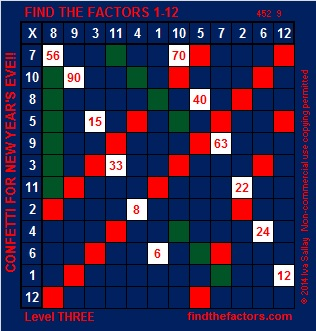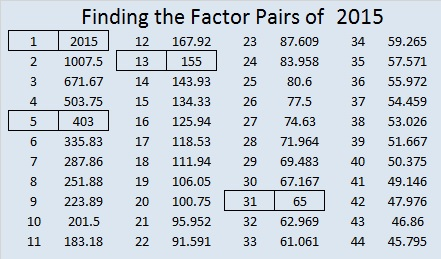# 337 What Will Be the Factors of 2015?

Since this is my 337th post, I’ll first give some information about the number 337, then I will predict the factors for 2015.

• 337 is a prime number.
• Prime factorization: 337 is prime.
• The exponent of prime number 337 is 1. Adding 1 to that exponent we get (1 + 1) = 2. Therefore 337 has exactly 2 factors.
• Factors of 337: 1, 337
• Factor pairs: 337 = 1 x 337
• 337 has no square factors that allow its square root to be simplified. √337 ≈ 18.358How do we know that 337 is a prime number? If 337 were not a prime number, then it would be divisible by at least one prime number less than or equal to √337 ≈ 18.358. Since 337 cannot be divided evenly by 2, 3, 5, 7, 11, 13, or 17, we know that 337 is a prime number.

Celebrate the New Year by giving this puzzle a try!

Print the puzzles or type the factors on this excel file: 12 Factors 2014-12-29On New Year’s Eve 2013 I predicted that the positive factors for 2014 would be 1, 2, 19, 38, 53, 106,1007, and 2014, and my predictions were 100% accurate!

On this last day of 2014, I boldly announce my predictions for the factors of the year 2015:

• The positive factors for 2015 will be 1, 5, 13, 31, 65, 155, 403, and 2015
• Some of these factors will occur in pairs: 1 and 2015, 5 and 403, 13 and 155, as well as 31 and 65.
• Unfortunately there will be some negative factors in 2015 as well. They will be -1, -5, -13, -31, -65, -155, -403, and -2015.

Whatever life throws your way, I wish you a happy, healthy, and prosperous 2015.This site uses Akismet to reduce spam. Learn how your comment data is processed.